# C Program to Convert Octal to Binary

In this article, we will learn how to create a program in C that converts any given octal number entered by the user at run-time into its equivalent binary value. At last, we have also created a function-driven program that does the same job. In a function-driven program, we have defined a function named OctToBin() that takes an octal number as an argument and converts it into its equivalent binary value.

But before going through the program, if you are not aware of

• Octal Number
• Binary Number
• Octal to Binary Conversion Process

then refer to the step-by-step Octal to Binary conversion procedure. Now let's move on to the program.

## Octal to binary in C

To convert an octal number to a binary number in C programming, you have to ask the user to enter an octal value and convert it into a binary value, then display the equivalent value in binary as an output. The question is: write a program in C that converts any given number (in the octal number system) to its equivalent value in the binary number system. The answer to this question is:

```#include<stdio.h>
#include<conio.h>
int main()
{
int octnum, rev=0, rem;
printf("Enter any Octal Number: ");
scanf("%d", &octnum);
while(octnum!=0)
{
rem = octnum%10;
rev = (rev*10) + rem;
octnum = octnum/10;
}
octnum = rev;
printf("\nEquivalent Binary value = ");
while(octnum!=0)
{
rem = octnum%10;
switch(rem)
{
case 0: printf("000");
break;
case 1: printf("001");
break;
case 2: printf("010");
break;
case 3: printf("011");
break;
case 4: printf("100");
break;
case 5: printf("101");
break;
case 6: printf("110");
break;
case 7: printf("111");
break;
default: printf(" InvalidOctalDigit(%d) ", rem);
break;
}
octnum = octnum/10;
}
getch();
return 0;
}```

As the above program was written in the Code::Blocks IDE, here is the sample run after a successful build and run. This is the first snapshot: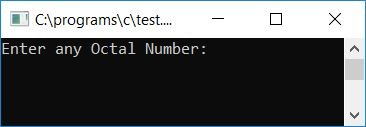Now supply any number, say 734, as input and press the ENTER key to see its value in binary form, as shown in the second snapshot of the sample run given here: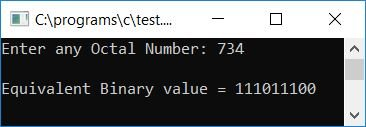As the number 734 has 3 digits, those are 7, 3, and 4. Here, 7 in binary is 111, 3 in binary is 011, and 4 in binary is 100. Therefore, after combining all numbers in octal and binary, we have 734 (in octal) equaling 111011100 (in binary), as shown in the above snapshot. It is also possible to write (734)8 = (111011100)2.

#### Program Explained

• Receive any number (in octal) as input, say 734.
• Now reverse the number using the while loop.
• Here we have reversed the octal number by finding the remainder one by one. The remainder gives the digit at the end. That is, the first remainder will give the last digit; then, divide the number by 10, and again find the remainder, which will give the second last digit, and so on. In this way, the first remainder becomes the first digit of the reverse number. For example, using the modulus (%) operator to find a digit or remainder of any number, say 734, That is, using 734%10, we will get 4 as the first digit or remainder. And here is the last digit of the number 734. Therefore, we have to reverse the number first.
• Now initialize the reverse of the number stored in the rev variable to the octnum variable.
• Create a while loop that runs until the value of octnum becomes 0.
• At first run, the condition of the while loop, octnum!=0 or 437!=0 (as we have reversed the number 734) evaluates to true, therefore program flow goes inside the loop.
• And octnum%10 or 437%10 or 7 (the first digit of 734) gets initialized to rem.
• Create a switch() case to check what the value present inside the rem variable is.
• Whatever the value present in the rem variable is, print its equivalent binary value. For example, if rem holds 7, then the binary equivalent of 7 gets printed as output, and after printing the binary equivalent of rem, octnum/10 or 437/10 or 43 gets initialized to octnum, and the program flow goes back to the condition of the while loop.
• There, the condition octnum!=0 or 43!=0 evaluates to true again, so the program flow goes inside the loop.
• And octnum%10 or 43%10 or 3 gets initialized to rem, and then, using switch(), the binary equivalent of 3 gets printed as output, and you continue to do the similar operation as told above, until the value of octnum becomes 0.
• In this way, the binary equivalent of a given octal number gets printed on the output screen.

### Octal to binary in C using the strcat() function

Let's make the same purpose program in a different way. Here we have used the string function named strcat() of the string.h library to concatenate the strings one by one. We have stored binary values in string format, as shown in the program given below. The question is, "Write a program in C that converts an octal number to its equivalent binary number using the library function strcat()." The answer to this question is:

```#include<stdio.h>
#include<conio.h>
#include<string.h>
int main()
{
int octnum, rev=0, rem, count=0;
char binnum = "";
printf("Enter any Octal Number: ");
scanf("%d", &octnum);
while(octnum!=0)
{
rem = octnum%10;
if(rem>7)
{
count++;
break;
}
rev = (rev*10) + rem;
octnum = octnum/10;
}
if(count==0)
{
octnum = rev;
printf("\nEquivalent Binary value = ");
while(octnum!=0)
{
rem = octnum%10;
switch(rem)
{
case 0: strcat(binnum, "000");
break;
case 1: strcat(binnum, "001");
break;
case 2: strcat(binnum, "010");
break;
case 3: strcat(binnum, "011");
break;
case 4: strcat(binnum, "100");
break;
case 5: strcat(binnum, "101");
break;
case 6: strcat(binnum, "110");
break;
case 7: strcat(binnum, "111");
break;
}
octnum = octnum/10;
}
printf("%s", binnum);
}
else
printf("\nInvalid Octal Digit %d", rem);
getch();
return 0;
}```

Here is the final snapshot of the above program's sample run: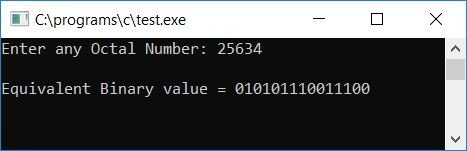As an octal digit cannot exceed a value greater than 7, we have used the count variable to check whether any digit of the given octal number exceeds the value 7 or not. If it exceeds, then its value gets incremented and it exits from the current loop. And before converting octal to binary, we checked whether count held its original value or not. If it holds, then all the octal digits are correct; otherwise, print any message like "An invalid octal digit was entered by you."

### Octal to Binary in C using Indirect Conversion

The question is, Write a program in C that converts an octal number to its equivalent binary number in such a way that the given octal number is first converted into a decimal number, and then the decimal number is converted into a binary number. The answer to this question is:

```#include<stdio.h>
#include<conio.h>
int main()
{
int octnum, decnum=0, binnum, rem, mul=1, i=0, count=0;
printf("Enter any Octal Number: ");
scanf("%d", &octnum);
while(octnum!=0)
{
rem = octnum%10;
if(rem>7)
{
count++;
break;
}
decnum = decnum + (rem*mul);
mul = mul*8;
octnum = octnum/10;
}
if(count==0)
{
while(decnum!=0)
{
binnum[i] = decnum%2;
i++;
decnum = decnum/2;
}
printf("\nEquivalent Binary Value = ");
for(i=(i-1); i>=0; i--)
printf("%d", binnum[i]);
}
else
printf("\nInvalid Octal Digit %d", rem);
getch();
return 0;
}```

Here is the final snapshot of the above program's sample run: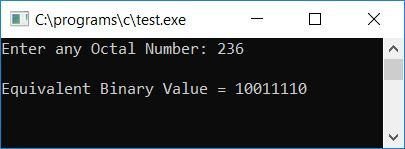### Octal to Binary in C using a User-Defined Function

To convert octal to binary using a user-defined function named OctToBin(). Create this function in such a way that it takes one argument that will be an octal number given by the user.

Here we have declared all the common variables (present inside both the functions main() and OctToBin()) as global variables to make them known through both functions. The variables i and count gets declared as static variables so that they can remember their previous values. As we all know, the static variable holds 0 as its initial value automatically. Therefore, we have not initialized zero to both the static variables i and count.

```#include<stdio.h>
#include<conio.h>
void OctToBin(int oct);
static int i, count;
int bin;
int main()
{
int octnum;
printf("Enter any Octal Number: ");
scanf("%d", &octnum);
OctToBin(octnum);
if(count==0)
{
printf("\nEquivalent Binary Value = ");
for(i=(i-1); i>=0; i--)
printf("%d", bin[i]);
}
else
printf("\nYou've entered invalid Octal Digit");

getch();
return 0;
}
void OctToBin(int oct)
{
int dec=0, rem, mul=1;
while(oct!=0)
{
rem = oct%10;
if(rem>7)
{
count++;
break;
}
dec = dec + (rem*mul);
mul = mul*8;
oct = oct/10;
}
if(count==0)
while(dec!=0)
{
bin[i] = dec%2;
i++;
dec = dec/2;
}
}```

Here is the final snapshot of the sample run: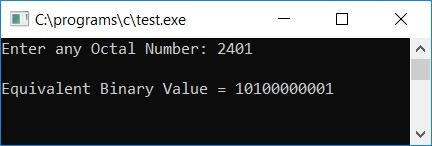#### The same program in different languages

C Quiz

« Previous Program Next Program »